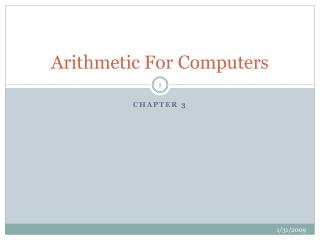DownloadDownload PresentationArithmetic For Computers

# Arithmetic For Computers

Download Presentation## Arithmetic For Computers

- - - - - - - - - - - - - - - - - - - - - - - - - - - E N D - - - - - - - - - - - - - - - - - - - - - - - - - - -
##### Presentation Transcript

1. Arithmetic For Computers Chapter 3

2. Topics for discussion • Number system: { radix/base, a set of distinct digits, operations} • Radix conversion • ASCII versus binary representation • Signed arithmetic; sign extension • Bounds check; validation • Addition and subtraction algorithms • Multiplication algorithms • Floating point representation • Floating point arithmetic algorithms

3. Number system • Radix or Base: 10 for decimal system, 2 for binary system, 8 for octal , 12 for duo-decimal (to count dozens), 16 for hexa-decimal • Decimal digits: {0,1,2,3,4,5,6,7,8,9} • Binary {0,1}: binary digit is a “bit” • Octal {0,1,2,3,4,5,6,7} • Hex {0,..9, A, B, C, D, E, F} • If we assume digits are number from right to left starting from 0th digit as the least significant digit (Little Endian), the value of the ith digit d in is: d x basei Example: Your pay is \$101 per hour. Would you prefer it (the base) in decimal, octal, hexadecimal or binary?

4. Number Representation • On the keyboard it is represented by ASCII: American Standard Code for Information Interchange: 7 bit code represented by a byte container. • For character representation this is a nice system. • How efficient is this representing numbers for processing? • Consider 4 ASCII digits. What is the range of integers you can represent with this? • 0 – 9999 • 4 X 8 = 32 bits • With 32 bits and binary systems and only positive numbers: • 0 – (232 -1). What is this value? Approx: 4,000,000,000 or 4G !

5. Signed Numbers • When we allow negative and positive numbers, half the range is occupied by positive numbers and the other by negative numbers. • How to represent the sign? Using a bit ? 0 for positive and 1 for negative? • Then with 32 bits: + 0 to +(231 -1) positive range - 0 to –(231 -1) negative range • A better representation for negative number is 2’s complement. How to compute 2’s complement? • What’s the advantage of 2’s complement? • Subtraction is equivalent to adding 2’s complement of the second operand. • Sign extension can be used to extend the number from, from 16 bits to 32 bits for example.

6. Dealing with Overflow

7. Dealing with overflow • Signed operations that result in overflow cause an exception whereas unsigned operations on overflow do not cause exception. • Example: • add, addi, sub will cause exception on overflow • addu, addiu, and subu will not cause exception on overflow • How to detect overflow in unsigned then? addu \$t0,\$t1,\$t2 xor \$t3,\$t1,\$t2 # check if signs differ slt \$t3,\$t3,\$zero # signs differ? bne \$t3,\$zero,No_Overflow # signs differ nop # signs area same xor \$t3,\$t0,\$t1 # find sign of sum slt \$t3,\$t3,\$zero # if sum sign is diff bne \$t3,\$zero,Overflow

8. Branches and Jumps • beq \$s1,\$s2,lab1 # if \$s1 == \$s2 jump to label lab1 • bne \$s1,\$s2,lab2 # if \$s1 ≠ \$s2 jump to label lab2 • j lab3 # unconditional jump to lab3 • jal proc3 # jump and link to proc3 • jr retAddr # jump back to callee • beqz \$s2, lab4 # is \$s2 == 0 then jump to lab4